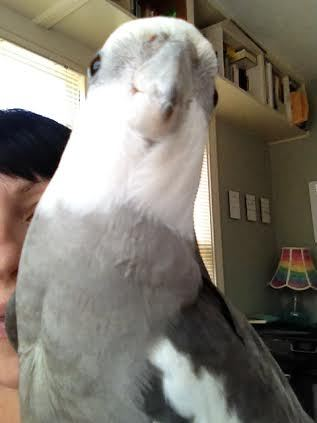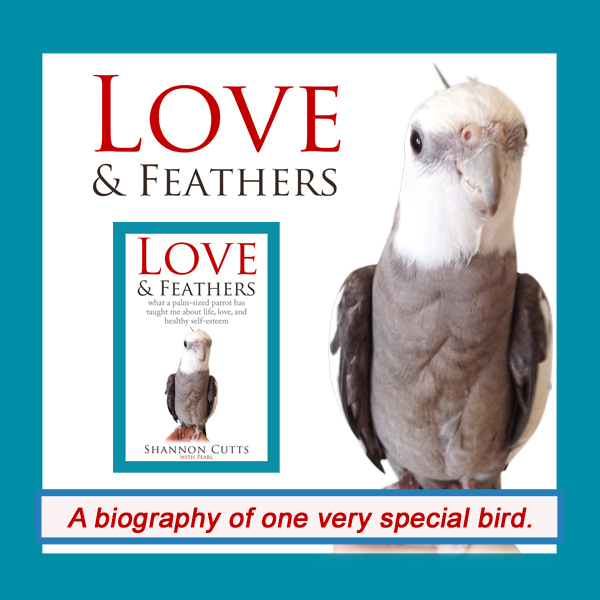# June 2018

 table div table+table+table div table{width:100%;padding:0}table div table+table+table div table img{width:96.23%;padding:0;float:none}table div table+table+table div table td{width:100%;padding:0 1.88% 18px}/* styles *//* styles */ Receiving this email as a forward? Sign up to keep following our little flock's adventures!
 table div table+table+table+table+table+table div table{width:100%;padding:0}table div table+table+table+table+table+table div table img{width:96.23%;padding:0;float:none}table div table+table+table+table+table+table div table td{width:100%;padding:0 1.88% 18px}/* styles */## The Joy of Taking Up SpacePearl, my 18-year-old parrot, eagerly claims any and all available space whenever the small rectangular flashing device comes out!

We all take up space. I take up a certain amount of space. My parrot, Pearl, takes up space as well - albeit less space than I take up, although he doesn't seem to realize this.

My nearly four-year-old tortoise, Malti, takes up more space than she realizes. On a rather consistent basis, Malti assesses whether she can fit into a space she wants to explore based on whether her head will fit inside it.

She then runs into problems of the sort that only arise when you have a big domed shell on your back that you can't see and thus don't realize is there.

Thinking back to when I was little, I don't remember feeling that taking up space was any type of issue. I don't even think I knew I took up space!

But then I started to get bigger. And as I got bigger than all my classmates (both taller and broader) other people started to notice. And then they started to make comments to make sure I noticed this size disparity as well.

All of a sudden I became very aware of taking up space. I also became concerned I was taking more than my share of available space. Then I started feeling anxious about how the space I was taking up seemed to really be bothering those around me - adults, teachers, peers.

Seen from that light (and the full perspective of hindsight), of course it made sense that I would start trying to become smaller so that I could take up less space. Others' comments about my appearance and general girth and height and overall size let me know in no uncertain terms that I wasn't supposed to be inhabiting as much space as I was taking up.

So I resolved to fix it. Looking back now, I can see that my naturally empathic, giving personality really wanted to make sure everything was equal for everybody. I can also see that my still immature brain and pre-teen thinking patterns automatically assumed that if I took up more space than I should be taking up, someone else was going to have to cope with less space than they needed and deserved.

This felt very wrong and I wanted to fix it as quickly as possible!

But now, after a 20-year battle with an eating disorder and another decade spent recovering from that, I now know what I was reacting to is the same thing scientists and statisticians call a "correlation."

A correlation is when two things occur at the same time but are not related to each other.

This is very different from causation, which is when two things occur at the same time and they are related to one another, as in one causes or influences the other or both inter-react in some way.

What I was experiencing was a correlation. I was taking up increasing amounts of space and people were commenting on it. So I automatically assumed the reason was a space deficit when the real reason was bullying and peer pressure and sometimes just plain old fashioned meanness.

I didn't realize at the time that there are no set space limitations for a human being. I had no idea no one else around me was really having to do without because I was greedily taking up my own space and part of someone else's space too.

Only recently have I really started to realize the impact this early misunderstanding about available space had on my whole life! It spoke to a scarcity mentality that has permeated nearly every area of my 47-year-old life to date.

Somehow, out of that early belief that I shouldn't be taking up so much space, I also came to believe I shouldn't have more than the bare bones of what I absolutely needed in any area of my life - from money to affection to friendship to health to happiness.

It even trickled down to how I live. Don't get me wrong - I am somewhat of an aspiring minimalist today for reasons that have more to do with carbon footprints and tiny houses than peer pressure or low self-esteem.

But certainly in my early days of downsizing, it felt like a relief to give away, donate or just dispose of anything I had in my possession that I wasn't using. Someone else perhaps needed it or could make better use of it than I could.

Today, I am coming down off of a period of my life when I reached the heaviest weight I have been as an adult. In other words, for a period of a year or so, I took up more space than I have ever taken in my whole life. Once again, my weight was an outward sign of inward imbalance, a red flashing warning light that something within was very wrong.

It was also the first experience in my life of simply not having any control over what my body was doing or how much space it was taking up. I was definitely attempting to lose this excess weight, but it wasn't coming off. My body was taking up that space whether I liked it or wanted to or not, and so I was being forced to make some level of peace with inhabiting more space than I felt comfortable claiming.

If I wanted to be here, that was the amount of space I needed, end of story.

Now, as my thyroid and I work together to heal ourselves and the extra pounds begin to smooth away once again, I am finding my feet at last in a space that I know is uniquely for me. It feels like the right shape and size for me.

I am not always squirming around in it, struggling to make all of me fit.

Neither am I rattling around in a roomier space than I feel I can cope with, wondering what to do with the excess.

Today, for the first time ever, I feel a level of peace with the space - the shape and size - of me. And it feels really, really GOOD.

With great respect and love,

Shannon

 table div table+table+table+table+table+table+table+table+table div table{width:100%;padding:0}table div table+table+table+table+table+table+table+table+table div table img{width:96.23%;padding:0;float:none}table div table+table+table+table+table+table+table+table+table div table td{width:100%;padding:0 1.88% 18px}/* styles */## How I enjoy taking up space today:

▪ By standing up straight with my shoulders down and back (yogis, you get it)!
▪ By coloring my hair blue, purple, platinum - or all three!
▪ By being very mindful of personal space - my own and others - on and off the road.
▪ By keeping my commitments to myself, whether they help pay the rent or not.
▪ By continually making the tough choice to change things up when something about my living space, daily routine, way of doing business or simply way of living begins to not feel quite right.
 ▪ By standing up straight with my shoulders down and back (yogis, you get it)!
 ▪ By coloring my hair blue, purple, platinum - or all three!
 ▪ By being very mindful of personal space - my own and others - on and off the road.
 ▪ By keeping my commitments to myself, whether they help pay the rent or not.
 ▪ By continually making the tough choice to change things up when something about my living space, daily routine, way of doing business or simply way of living begins to not feel quite right.
 table div table+table+table+table+table+table+table+table+table+table+table div table{width:100%;padding:0}table div table+table+table+table+table+table+table+table+table+table+table div table img{width:96.23%;padding:0;float:none}table div table+table+table+table+table+table+table+table+table+table+table div table td{width:100%;padding:0 1.88% 18px}/* styles */# Get your copy of Love & Feathers the book!Click on the image of Pearl to order your signed copy!Pearl will even beak-o-graph it for you!
 table div table+table+table+table+table+table+table+table+table+table+table+table+table+table+table+table div table{width:100%;padding:0}table div table+table+table+table+table+table+table+table+table+table+table+table+table+table+table+table div table img{width:96.23%;padding:0;float:none}table div table+table+table+table+table+table+table+table+table+table+table+table+table+table+table+table div table td{width:100%;padding:0 1.88% 18px}/* styles */# Only Malti Could Pull Off This Look!!

 table.module-17{width:75.47%;padding:0}table div table+table+table+table+table+table+table+table+table+table+table+table+table+table+table+table+table+table div table{width:75.47%;float:none;margin-left:auto;margin-right:auto;padding:0}table div table+table+table+table+table+table+table+table+table+table+table+table+table+table+table+table+table+table div table a{border:0 none;text-decoration:none}table div table+table+table+table+table+table+table+table+table+table+table+table+table+table+table+table+table+table div table img{width:100%!important;border:0 none;text-decoration:none}table div table+table+table+table+table+table+table+table+table+table+table+table+table+table+table+table+table+table div table td{width:100%;padding:0}/* styles */
 table div table+table+table+table+table+table+table+table+table+table+table+table+table+table+table+table+table+table+table+table div table{width:100%;padding:0}table div table+table+table+table+table+table+table+table+table+table+table+table+table+table+table+table+table+table+table+table div table img{width:96.23%;padding:0;float:none}table div table+table+table+table+table+table+table+table+table+table+table+table+table+table+table+table+table+table+table+table div table td{width:100%;padding:0 1.88% 18px}/* styles */# Bruce on the Blue (Cozy) Carpet!

 table.module-21{width:75.47%;padding:0}table div table+table+table+table+table+table+table+table+table+table+table+table+table+table+table+table+table+table+table+table+table+table div table{width:75.47%;float:none;margin-left:auto;margin-right:auto;padding:0}table div table+table+table+table+table+table+table+table+table+table+table+table+table+table+table+table+table+table+table+table+table+table div table a{border:0 none;text-decoration:none}table div table+table+table+table+table+table+table+table+table+table+table+table+table+table+table+table+table+table+table+table+table+table div table img{width:100%!important;border:0 none;text-decoration:none}table div table+table+table+table+table+table+table+table+table+table+table+table+table+table+table+table+table+table+table+table+table+table div table td{width:100%;padding:0}/* styles */
 table div table+table+table+table+table+table+table+table+table+table+table+table+table+table+table+table+table+table+table+table+table+table+table+table div table{width:100%;padding:0}table div table+table+table+table+table+table+table+table+table+table+table+table+table+table+table+table+table+table+table+table+table+table+table+table div table img{width:96.23%;padding:0;float:none}table div table+table+table+table+table+table+table+table+table+table+table+table+table+table+table+table+table+table+table+table+table+table+table+table div table td{width:100%;padding:0 1.88% 18px}/* styles */## Meet Pearl, Malti, Bruce & Me

 table div table+table+table+table+table+table+table+table+table+table+table+table+table+table+table+table+table+table+table+table+table+table+table+table+table+table div table,table.module-25{width:34.34%;float:left;padding:0}table div table+table+table+table+table+table+table+table+table+table+table+table+table+table+table+table+table+table+table+table+table+table+table+table+table+table div table a{border:0 none;text-decoration:none}table div table+table+table+table+table+table+table+table+table+table+table+table+table+table+table+table+table+table+table+table+table+table+table+table+table+table div table img{width:100%!important;border:0 none;text-decoration:none}table div table+table+table+table+table+table+table+table+table+table+table+table+table+table+table+table+table+table+table+table+table+table+table+table+table+table div table td{width:100%;padding:0 20px 20px 0}/* styles */ Hi! It is so good to meet you here each month! I'm so excited about our new book, "Love & Feathers: what a palm-sized parrot has taught me about life, love, and healthy self-esteem."The book focuses on the power of animal mentors in our lives and features my parrot, Pearl. Malti is a young red-foot tortoise with a healthy appetite for food, snoozing and life! Bruce, a rescued Texas 3-toed box turtle, is our newest shelled flock member. My first book, "Beating Ana: how to outsmart your eating disorder and take your life back," shares how I recovered from an eating disorder so I could pursue my creative dreams. MentorCONNECT, the first global eating disorders nonprofit mentoring community, emerged from the experience of writing "Beating Ana." While we closed in April 2017, the website continues to provide information about mentoring-based recovery support resources. ==> To connect with me: www.shannoncutts.com ==> To connect with Pearl, Malti & Bruce: www.loveandfeathersandshells.com
 table div table+table+table+table+table+table+table+table+table+table+table+table+table+table+table+table+table+table+table+table+table+table+table+table+table+table+table div table{width:100%;padding:0}table div table+table+table+table+table+table+table+table+table+table+table+table+table+table+table+table+table+table+table+table+table+table+table+table+table+table+table div table img{width:96.23%;padding:0;float:none}table div table+table+table+table+table+table+table+table+table+table+table+table+table+table+table+table+table+table+table+table+table+table+table+table+table+table+table div table td{width:100%;padding:0 1.88% 18px}/* styles */table div table+table+table+table+table+table+table+table+table+table+table+table+table+table+table+table+table+table+table+table+table+table+table+table+table+table+table+table div table td,table.module-27{width:100%;padding:0}table div table+table+table+table+table+table+table+table+table+table+table+table+table+table+table+table+table+table+table+table+table+table+table+table+table+table+table+table div table{width:100%;float:none;margin-left:auto;margin-right:auto;padding:0}table div table+table+table+table+table+table+table+table+table+table+table+table+table+table+table+table+table+table+table+table+table+table+table+table+table+table+table+table div table a{border:0 none;text-decoration:none}table div table+table+table+table+table+table+table+table+table+table+table+table+table+table+table+table+table+table+table+table+table+table+table+table+table+table+table+table div table img{width:100%!important;border:0 none;text-decoration:none}/* styles */
 table.module-28{width:74.15%;padding:0}table div table+table+table+table+table+table+table+table+table+table+table+table+table+table+table+table+table+table+table+table+table+table+table+table+table+table+table+table+table div table{width:74.15%;float:none;margin-left:auto;margin-right:auto;padding:0}table div table+table+table+table+table+table+table+table+table+table+table+table+table+table+table+table+table+table+table+table+table+table+table+table+table+table+table+table+table div table a{border:0 none;text-decoration:none}table div table+table+table+table+table+table+table+table+table+table+table+table+table+table+table+table+table+table+table+table+table+table+table+table+table+table+table+table+table div table img{width:100%!important;border:0 none;text-decoration:none}table div table+table+table+table+table+table+table+table+table+table+table+table+table+table+table+table+table+table+table+table+table+table+table+table+table+table+table+table+table div table td{width:100%;padding:0}/* styles */
 table div table+table+table+table+table+table+table+table+table+table+table+table+table+table+table+table+table+table+table+table+table+table+table+table+table+table+table+table+table+table div table{width:100%;padding:0}table div table+table+table+table+table+table+table+table+table+table+table+table+table+table+table+table+table+table+table+table+table+table+table+table+table+table+table+table+table+table div table img{width:96.23%;padding:0;float:none}table div table+table+table+table+table+table+table+table+table+table+table+table+table+table+table+table+table+table+table+table+table+table+table+table+table+table+table+table+table+table div table td{width:100%;padding:0 1.88% 18px}/* styles */## Reprints & Reposts

REPRINTS: Would you like to use any portion of this material in your newsletter, blog or website? You may do so as long as you also include this copyright information: